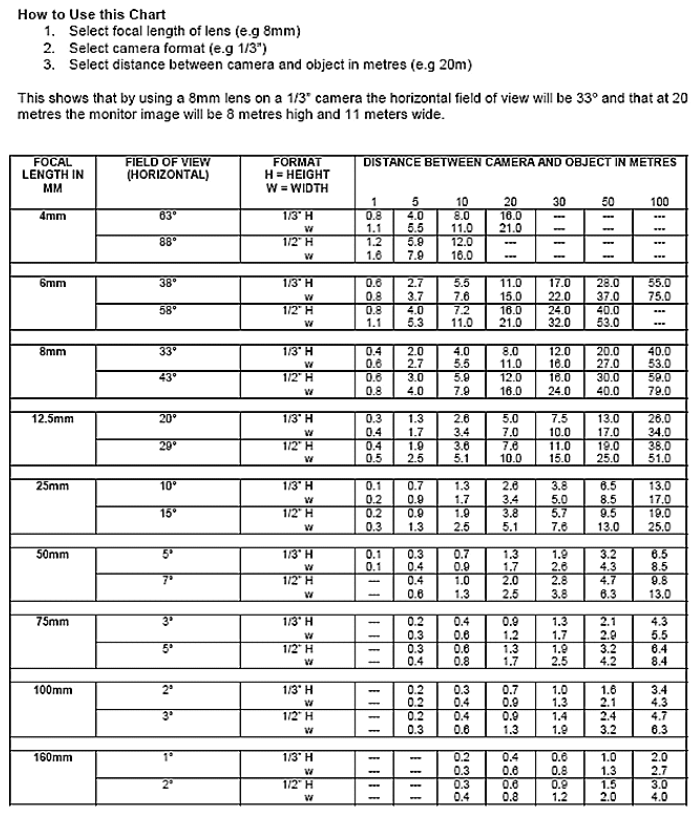### CCTV Lens Calculator

For a quick 1/3″ CCD Camera you can work out the lens required using this simple method:
Distance from object multiplied by 4.8, divided by horizontal or vertical area equals the lens required.

d x 4.8 / A = L

e.g.
To focus on an area 5m wide from a camera installed 20m away would be:

20 x 4.8 / 5 = 19.2
So you would need a lens with a focal length of 19.2mm